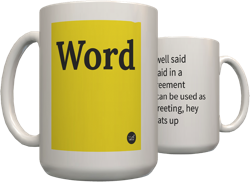Top definition
Letters, numbers, and/or symbols that seemingly appear out of no where in relation to given mathematical context.
voodoo math:
Find the equation of the tangent plane to the surface x^2z-y^3z^5+xy=9 at the point P=(1,2,-1).